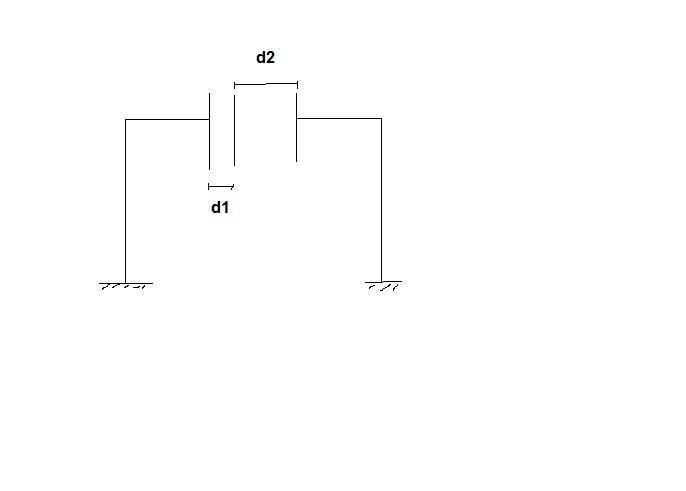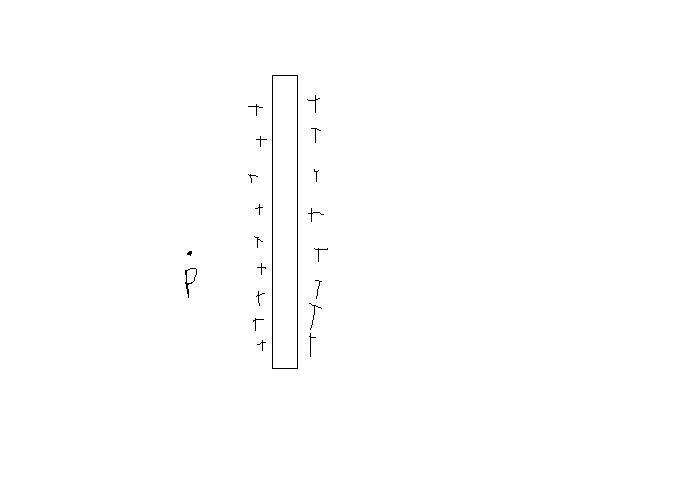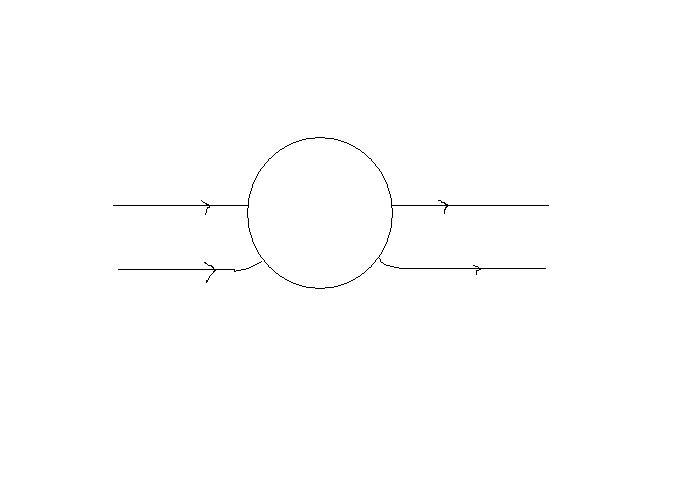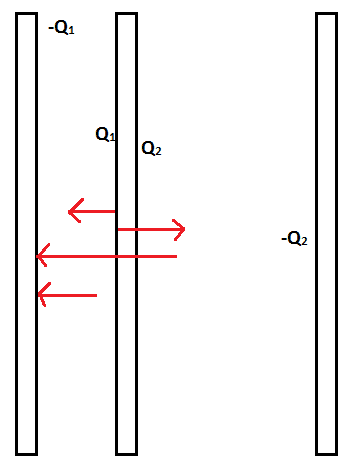# Charge on three metallic plates

zorro

## Homework Statement

3 metallic plates are placed at a separation d1 and d2 as shown in figure. A charge Q is given to the middle plate which is free to move, then

A)it will slide towards left if d2>d1
B)it will slide towards right if d2>d1## The Attempt at a Solution

I found out the charge distributions on the the first and last plate as -Qd2/d1+d2 and -Qd1/d1+d2 resp.

Now to calculate the force on the middle plate due to one plate (say first), shall I take the charge on the middle plate as Q or Qd2/d1+d2 ?

#### Attachments

cupid.callin

take it as Q ... net charges on plates will give force b/w the plates

zorro

Even I thought so.....but that does not give the correct answer XD

cupid.callin

does the other way around give correct answer?

zorro

Yeah

Homework Helper

Since the middle plate and the outer plates have opposite charges, the middle plate experiences a tug-of-war.

zorro

Yes it does, but why don't we take the net charge on the middle plate to find out the force of attraction acting on it due to other plates?
Are the charges on one side isolated from one plate?

Homework Helper

The charges sit on both surfaces of the inner plate. They feel the electric field built up in the empty space at their side. The charges are attached to particles of the plate, cannot leave it plate, so the plate feels the force due to the electric field at the left-hand side and a force of opposite sign from the right-hand electric field. The plate will slide in the direction of the resultant force.

ehild

zorro

They feel the electric field built up in the empty space at their side.

Refer this:(I lost my patience while drawing + signs)

The electric field at point P is due to the left and right side of the charged plate.

#### Attachments

Homework Helper

It is a metal plate, Q charge on both sides. When the area of the plate A is big the electric field is homogeneous near the plate and far from the edge at both sides. Apply Gauss' law. 2A*E=2Q/ε: The electric field strength is Q/(Aε) that is, the surface charge density/ε.
In terms of electric field lines, the electric field strength is the number of field lines crossing perpendicularly an unit area. For an isolated point charge Q, the number of field lines crossing the surface of a sphere of radius R is
Q/(4πεR2) * (4πR2) = Q/ε,
that is Q/ε field lines emerge from a positive point charge of magnitude Q. In case of a surface charge on a metal plate, all the field lines point outwards so E=Q/(Aε).
In your case tha surface charge density is different at the sides of the metal plate so the electric fields are different to. You can imagine the three plates as two different capacitors connected to the same potential.

ehild

Last edited:
zorro

I agree with that.
But in the problem, the middle plate has different charges on either side.
Consider a cylinderical Gaussian surface passing through the plate.
(E1+E2)A=(Enet)A=(Q1+Q2)/ε

i.e. The total electric field at any point in between the two regions on either side of the middle plate equal Q1 + Q2 = Q/ε

Proceeding this way I don't get the correct answer.

Homework Helper

Meanwhile I edited my post, read it. You assumed that the electric field is the same at both sides. Try to choose a Gaussian surface with one base inside the metal.

ehild

zorro

No I did not assume the fields to be same. That is why I used E1 and E2.
"Try to choose a Gaussian surface with one base inside the metal. "
That will give electric field only due to one of the sides of the plate (E1 or E2)

Homework Helper

What are E1 and E2? Is E1 the electric field at one side and E2 the electric field at the other side? Then what is E net?

(E1+E2)A=(Q1+Q2)/ε is correct, but it does not mean that E1=E2.

Gauss'law states that the electric flux through a closed surface equals to the enclosed charge divided by ε. It does not matter how much charges are outside. You know that the electric field is zero in the metal. Choosing the closed surface with one base inside the metal and the other base just outside, the total flux is A*E1 =ε Q1. The same is valid for the other side: A*E2=ε Q2. So E1=Q1/ε and E2=Q2/ε.

zorro

Forget Gauss' theorem for a minute.
Refer the figure in #9.
Consider the charges to be different on both sides.
Now at the point P, is there any electric field due to the charges on the other side of the plate?

Homework Helper

The electric field lines do not penetrate inside the metal. There is no electric field at one side originating from the surface charges on the other side.

ehild

zorro

I think that 'penetrate' is not the right word here. Its just that the field lines don't exist inside a metal/conductor.
Consider a situation where a charge q is placed at the centre of an uncharged spherical metallic hollow shell. There are no field lines inside the shell but they are present outside the shell!

Consider this situation too-The field lines come out from the other side though they don't exist inside.

What I am trying to say is that the plate in our case should not shield the electric field due to charges on one side.

#### Attachments

Last edited:
Staff Emeritus
Homework Helper
Gold Member

Assuming that they can thought of as parallel plate capacitors with the same plate area, A, C1=Aε0/d1 and C2=Aε0/d2.

Both capacitors are grounded on one side. The potential difference across each capacitor is the same.

$$V=\frac{Q_1}{C_1}\ \text{ and }\ V=\frac{Q_2}{C_2}\,,\text{ where }Q_1+Q_2=Q$$

That should allow you to compare the charge on each side of the common metal plate, and thus compare the electric field on each side.

zorro

Why would I name the thread 'Capacitors' without knowing anything about them?Assuming that they can thought of as parallel plate capacitors with the same plate area, A, C1=Aε0/d1 and C2=Aε0/d2.

Both capacitors are grounded on one side. The potential difference across each capacitor is the same.

$$V=\frac{Q_1}{C_1}\ \text{ and }\ V=\frac{Q_2}{C_2}\,,\text{ where }Q_1+Q_2=Q$$

That should allow you to compare the charge on each side of the common metal plate, and thus compare the electric field on each side.

I already found out the charge distribution. My problem is something else (refer previous posts).

Staff Emeritus
Homework Helper
Gold Member

E1=V/d1 & E2=V/d2

zorro

Yes that comes out using formulae.
But I am thinking about the problem another way. Why is the electric field between the first two plates only due to the charge on the middle plate on one side and not due to the other side? The electric field due to the charges on the other side cannot be shielded by a metallic plate (Why? - refer post #17)

Last edited:
cupid.callin

Look at the pici have shown only for +-Q1 ... the field outside the region b/w them has no field as their fields gets cancelled as shown in fig.

hence there will be no force on Q2 due to -Q1

#### Attachments

Mentor

In the absence of external plates, if you put a charge Q on a single thin plate, the charge spreads uniformly on both sides of the plate. What is the electric field near a side of the plate?

Next consider a neutral plate and you bring near it (to some distance d) a second plate carrying a charge Q. What is the disposition of charges on the neutral plate?

Bring another charged plate near the other side of the neutral plate, again with charge Q and this time distance d2. What's the distribution of charges on the neutral plate?

Now use superposition.

zorro

@cupid.callin
I don't understand your figure totally but one comment-
Q1 and Q2 are different.......the field does not get cancelled.

cupid.callin

the fields i have shown are only due to -Q1 n Q1
if Q2 was supposed to cancel the field then that means that there is field at position of Q2 ... then why there would be a question ... the fields of "" ---Q1 and -Q1 --- "" at position of Q2 are cancelled ... thats why q2 experience no force

zorro

In the absence of external plates, if you put a charge Q on a single thin plate, the charge spreads uniformly on both sides of the plate. What is the electric field near a side of the plate?

its Q/Aε (if A=area of the plates)

Next consider a neutral plate and you bring near it (to some distance d) a second plate carrying a charge Q. What is the disposition of charges on the neutral plate?

Bring another charged plate near the other side of the neutral plate, again with charge Q and this time distance d2. What's the distribution of charges on the neutral plate?

Now use superposition.

The distance between the plates does not affect the distribution of charges (as long as it is not infinite). The neutral plate remains neutral with 0 charge on both sides.

zorro

the fields i have shown are only due to -Q1 n Q1
if Q2 was supposed to cancel the field then that means that there is field at position of Q2 ... then why there would be a question ... the fields of "" ---Q1 and -Q1 --- "" at position of Q2 are cancelled ... thats why q2 experience no force

I have no idea what you are talking about. (btw your figure is deceptive- there is no field line inside a plate!)

Staff Emeritus
Homework Helper
Gold Member

Look in your textbook at how the author arrives at the field of a parallel plate capacitor.

zorro

That does not help. In the derivation, there is no charge on the outer sides of the two plates. That makes things easier.

Staff Emeritus
Homework Helper
Gold Member

That does not help. In the derivation, there is no charge on the outer sides of the two plates. That makes things easier.
The charge on the outside is (should be) outside of the Gaussian surface.

Mentor

its Q/Aε (if A=area of the plates)
It should be half that, because the charge is divided over two equal surfaces of area A, and the gaussian surface used contains only the charge on one face. The field inside the conductor is zero.

The distance between the plates does not affect the distribution of charges (as long as it is not infinite). The neutral plate remains neutral with 0 charge on both sides.

Look up "Charge-induced charge separation". The neutral plate will become polarized.

zorro

The charge on the outside is (should be) outside of the Gaussian surface.

The Gaussian surface can emerge from both sides of the plate as it has a continuous charge distribution. It is not discrete.

It should be half that, because the charge is divided over two equal surfaces of area A, and the gaussian surface used contains only the charge on one face. The field inside the conductor is zero.

Yeah I missed the 2.

Look up "Charge-induced charge separation". The neutral plate will become polarized.

Metallic plate gets polarized?? :surprised:

cupid.callin

why are you making such an easy question so complicated...

the capacitor made of the 1st plate and left side of middle plate has no field outside it ...... so i wont have any force on the charge on the right side of middle plate and and third plate

Mentor

Metallic plate gets polarized?? :surprised:

Yup. http://en.wikipedia.org/wiki/Electrostatic_induction" [Broken].

Last edited by a moderator:
cupid.callin

Metallic plate gets polarized?? :surprised:

yes because they have free electrons

even dielectrics gets polarized